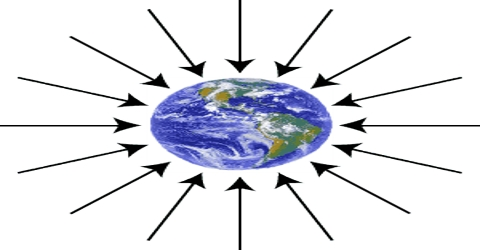Physics

# Gravitational FieldGravitational Field

Definition: Any region in which, if a body is placed gravitational force acts on it, then that region is called gravitational field. When the distance between two bodies is infinity, then attractive forte becomes zero. Theoretically gravitational field extends up to infinity.

The gravitational field is the gravitational force per unit mass that would be exerted on a small (so it doesn’t measurably affect the gravitational field) test mass at that point. It is a vector field, and points in the direction of the force that a small test mass would feel at that point.

For a point particle of mass MM, the magnitude of the resultant gravitational field strength g, at distance r from M, is;

g = GM/r2

It is measured in units of force per mass, usually newtons per kilogram (N/kg). A gravitational field is a type of force field and is analogous to electric and magnetic fields for electrically charged particles and magnets, respectively.

From the above discussion it is seen that the limit of force acting between two bodies extends up to a fixed space. The gravitational force between two bodies acts without any contact between them. This can be understood clearly from the idea of gravitational field. According to this idea, area around a body acquires a special property because of the presence of the body. Because of this property if another body is brought to that area then gravitational field of that body acts on the body. So, gravitational field is a medium to communicate gravitational force and keeping it confined to a region it acts. That region is called gravitational held.